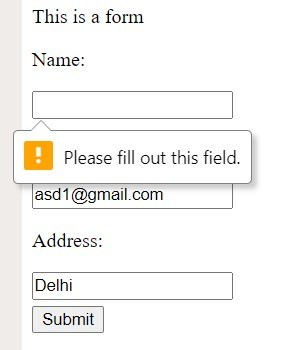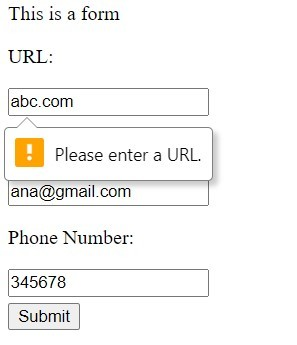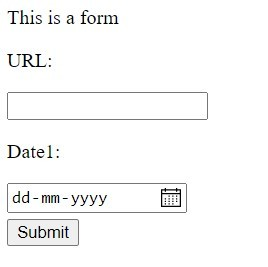Related Articles
How to create form validation by using only HTML ?
• Last Updated : 15 Apr, 2020

In Web Development, we often use JavaScript with HTML to validate the form, but we can also do the same via HTML in the following ways.

HTML <input> required Attribute: In input tag of HTML, we can specify via “required attribute”. It informs the browser (HTML5 supported) that the field can’t be left blank. Browsers vary in terms of this implementation, some browsers case a shadow to the box or some show a warning.

• Example:

 ` ` `<``html` `lang``=``"en"``> ` ` `  `<``head``> ` `    ``<``title``>Form ` ` ` ` `  `<``body``> ` `    ``<``p``>This is a form ` `    ``<``form``> ` `        ``<``p``>Name: ` `        ``<``input` `type``=``"text"` `required> ` `        ``<``p``>Email: ` `        ``<``input` `type``=``"email"` `required> ` `        ``<``p``>Address: ` `        ``<``input` `type``=``"text"` `required> ` `        ``<``br``> ` `        ``<``button` `style``=``"margin-top: 5px;"``> ` `          ``Submit ` `        `` ` `    `` ` ` `  ` ` ` `  ` `

• Output:HTML <input> type Attribute: In input tag, if we require for the user to input their email-id we can set the type attribute to email, the same is applicable to number, date or URL. Similar to the required attribute, different browsers have different implementations.

• Example:

 ` ` `<``html` `lang``=``"en"``> ` ` `  `<``head``> ` `    ``<``title``>Form ` ` ` ` `  `<``body``> ` `    ``<``p``>This is a form ` `    ``<``form``> ` `        ``<``p``>URL: ` `        ``<``input` `type``=``"url"``> ` `        ``<``p``>Email: ` `        ``<``input` `type``=``"email"``> ` `        ``<``p``>Phone Number: ` `        ``<``input` `type``=``"number"``> ` `        ``<``br``> ` `        ``<``button` `style``=``"margin-top: 5px;"``> ` `          ``Submit ` `        `` ` `    `` ` ` `  ` ` ` `  ` `

• Output:HTML <input> pattern Attribute: We already know, apart from using default rules we can also set our rules as for the pattern of URL, date or price, etc.

• Example:

 ` ` `<``html` `lang``=``"en"``> ` ` `  `<``head``> ` `    ``<``title``>Form ` ` ` ` `  `<``body``> ` `    ``<``p``>This is a form ` `    ``<``form``> ` `        ``<``p``>URL: ` `        ``<``input` `type``=``"url"` `pattern``=``"https?://.+"``> ` `        ``<``p``>Date1: ` `        ``<``input` `type``=``"date"`  `               ``pattern``=``"\d{2, 1}/\d{2, 1}/\d{4}"``> ` `        ``<``br``> ` `        ``<``button` `style``=``"margin-top: 5px;"``> ` `          ``Submit ` `        `` ` `    `` ` ` ` ` `  ` `

•My Personal Notes arrow_drop_up
Recommended Articles
Page :